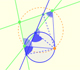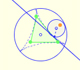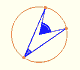The three points of intersection of the adjacent trisectors of the angles of any triangle are the vertices of an equilateral triangle called the Morley Triangle.

This was a surprising discovery made by Frank Morley (1899).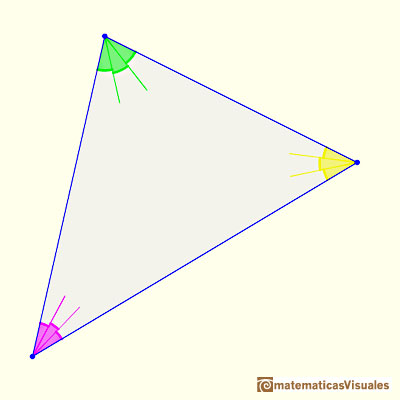Extend the trisections ...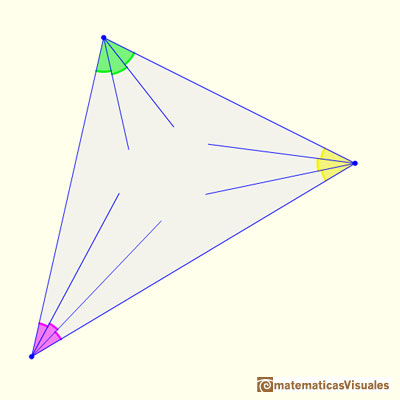We consider the three points of intersection of the adjacent trisectors ...And we always get an equilateral triangle.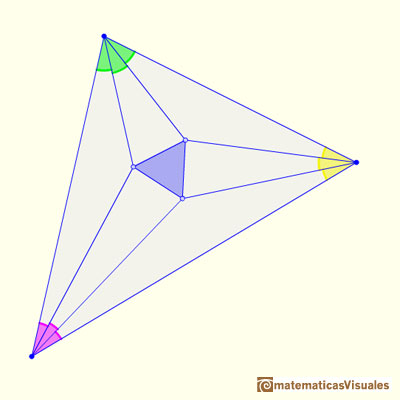In the near future I am going to publish an animation with John Conway's proof of Morley's Theorem.

REFERENCES

Coxeter, H. S. M. and Greitzer, S. L. Geometry Revisited. Washington, DC: Math. Assoc. Amer.
Coxeter, H. S. M. Introduction to Geometry, 2nd ed. New York: John Wiley and sons, 1969.